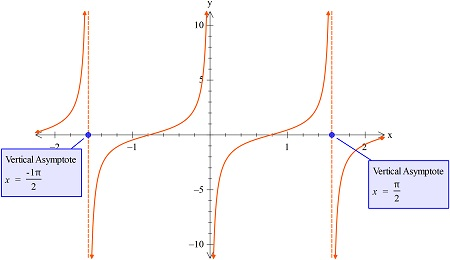# Find the period y = tan(2x - pi/2). Graph the function.

## Question:

Find the period {eq}y = \tan \left ( 2x - \frac{\pi}{2} \right ) {/eq}. Graph the function.

## Trigonometric Functions:

The function whose period is to be found will be found using the frequency known, or by comparing to the standard fo=unction and then comparing it with the period. the time period and frequency are related to each other.

Here the period {eq}y = \tan \left ( 2x - \frac{\pi}{2} \right ) {/eq}

is given by

{eq}T=\frac{2\pi }{2}=\pi {/eq}

So, this is because the coefficient of x is just 2 here.

Also, the graph of the function is plotted using the graphing utility as follows: